Courses

# Logical Reasoning - Test 10

## 25 Questions MCQ Test SSC CGL Tier 1 Mock Test Series | Logical Reasoning - Test 10

Description
This mock test of Logical Reasoning - Test 10 for SSC helps you for every SSC entrance exam. This contains 25 Multiple Choice Questions for SSC Logical Reasoning - Test 10 (mcq) to study with solutions a complete question bank. The solved questions answers in this Logical Reasoning - Test 10 quiz give you a good mix of easy questions and tough questions. SSC students definitely take this Logical Reasoning - Test 10 exercise for a better result in the exam. You can find other Logical Reasoning - Test 10 extra questions, long questions & short questions for SSC on EduRev as well by searching above.
QUESTION: 1

### A word is represented by only one set of numbers as given in any one of the alternatives. The sets of numbers given in the alternatives are represented by two classes of alphabets as shown in the given two matrices. The columns and rows of Matrix – I are numbered from 0 to 4 and that of Matrix – ll are numbered from 5 to 9. A letter from these matrices can be represented first by its row and next by its column, for example, 'F' can be represented by 32, 42 etc. and 'M' can be represented by 88, 68 etc. Similarly, you have to identify the set for the word ‘SNOW'.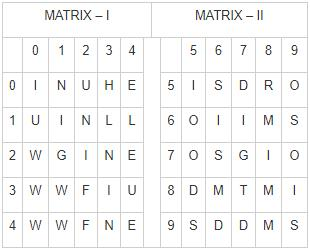Solution:

1) 76, 43, 59, 21 ⇒ S, N, O, G
2) 95, 23, 79, 10 ⇒ S, N, O, U
3) 99, 01, 57, 30 ⇒ S, N, D, W
4) 69, 12, 65, 20 ⇒ S, N, O, W
Therefore, “69, 12, 65, 20” are set of number which represent word “SNOW”.

QUESTION: 2

### Direction: In the following question, select the odd word from the given alternatives.

Solution:

ECS (Electronic Clearing Service) is used for making bulk payments, i.e. Transfer of money to multiple accounts.
RTGS (Real-time gross settlement) and NEFT (National Electronic Funds Transfer) are also ways to transfer money between bank accounts.
EMI (Equated Monthly Installments), on the other hand, is used to pay back an amount that has been loaned. This is done on a monthly basis.
Thus, EMI is not a form of transferring sums of money to other accounts, and hence is the odd one out.

QUESTION: 3

### Choose the odd number from the given alternatives.

Solution:

1) 48 ⇒ 42 × (4 – 1) = 16 × 3 = 48
2) 180 ⇒ 62 × (6 – 1) = 36 × 5 = 180
3) 18 ⇒ 32 × (3 – 1) = 9 × 2 = 18
4) 150 ⇒ 52 × (5 – 1) = 25 × 4 = 100
Hence, “150” is odd one among given alternatives.

QUESTION: 4

Directions: Select the related number from the given alternatives.
RUSTS: 96878∷STSRU: ?

Solution:

The letters R, S, T and U have codes 9, 8, 7 and 6 respectively.
Therefore the code for RUSTS would be 96878 while the code for STSRU would be 87896

QUESTION: 5

Identify the diagram that best represents the relationship among classes given below.
Engineer, Smoker, Indian, Non-Smoker

Solution:

→ Engineer can be either smoker or non-smoker and Indian.
→ Indian can be either smoker or non-smoker and Engineer.
→ Smoker can be an Indian and Engineer but he can’t be Non-smoker.
→ Non-smoker can be an Indian and Engineer but he can’t be Smoker.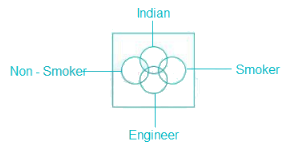Therefore, above image best express relationship between Engineer, Smoker, Indian, Non-Smoker.

QUESTION: 6

What should come in the place of question mark ? in the given number series?
12, 36, 33, 99, ?, 288

Solution:

The given series is as follows: x3, -3
12 x 3 = 36
36 – 3 = 33
33 x 3 = 99
99 – 3 = 96
96 x 3 = 288
Hence, 96 is the correct answer.

QUESTION: 7

Which one set of letters when sequentially placed at the gaps in the given letter series shall complete it?
_ b c d a _ c d a b _ d a b c _

Solution:

A.   d b c d a c c d a b a d a b c b
B.  c b c d a d c d a b a d a b c b
C.  a b c d a b c d a b c d a b c d
D.  c b c d a c c d a b d d a b c a
we can see that option c forms a pattern abcdabcdabcdabcd.

QUESTION: 8

Find the missing term in the given series.
AGMSY, CIOUA, EKQWC, ?, IOUAG, KQWCI

Solution:

The pattern followed is that in each term each letter is + 2 from its previous subsequent letter in previous term i.e.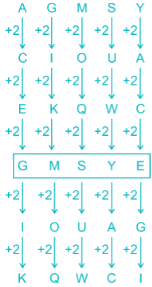QUESTION: 9

If HOPE is coded as 5 and HUMANS as 7, what is the code number for WALLETS?

Solution:

The code is the number of letters of the word + 1.
For HOPE, the number of letters is 4 → 4 + 1 = 5 = code for HOPE.
Similarly for HUMANS it is 6 + 1 =7 and for WALLETS it is 7 +1 =8.

QUESTION: 10

Neeraj is facing north, then he turns 45 degree right and goes 25 m, then turns in south – east direction to move 25 m and from there 25 m to east. In which direction/place is he from his original place?

Solution:

Direction diagram can be traced as information given in question,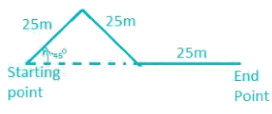Hence, Neeraj is in East with respect to his original position.

QUESTION: 11

In a drama, two characters are related to each other. One of them is the mother of the other’s daughter. How are the two related to each other?

Solution:

The character is the mother of the other character’s daughter. This means that they are both parents of the same child, and hence are husband and wife.

QUESTION: 12

If alphabets are serially numbered, one of the answer give below has a meaningful word hidden in it. Identify the answer.

Solution:

Converting to words:

1. 5, 18, 5, 8, 1, 3, 5 - EREHACE
2. 20, 5, 1, 3, 8, 5, 18 - TEACHER
3. 5, 1, 3, 5, 20, 8, 18 - EACETHR
4. 18, 5, 3, 8, 1, 5, 20 - RECHAET

Clearly, TEACHER is the only meaningful word.
Hence the answer is ‘20, 5, 1, 3, 8, 5, 18’.

QUESTION: 13

Find out the odd letters from the given alternatives.

Solution:

1) ⇒ B = 2; C = 3 ⇒ 2 + 3 = 5
2) IF ⇒ I = 9; F = 6 ⇒ 9 + 6 = 15
3) CL ⇒ C = 3; L = 12 ⇒ 12 + 3 = 15
4) GH ⇒ H = 8; G = 7 ⇒ 7 + 8 = 15
Therefore, “BC” is odd one out.

QUESTION: 14

In the following question, select the related word from the given alternatives.
Vacation : Holiday : : Vocation : ?

Solution:

Vacation: - a fixed holiday period between terms in universities and law courts.
Vocation:- a strong feeling of suitability for a particular career or occupation.
Hence, Vacation : Holiday : : Vocation : Career

QUESTION: 15

Which of the following cube in the answer figure cannot be made based on the unfolded cube in the question figure?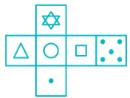Solution:

Opposite faces should not be together in the folded cube.
Triangle on left side is opposite to circle.
Triangle on top is opposite to single dot.
5 dots are opposite to the circle.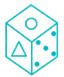Therefore, image in option 1) will be correct answer.

QUESTION: 16

Directions: In question, select the missing number from the given responses.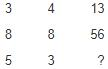Solution:

We can see a pattern when we move row wise: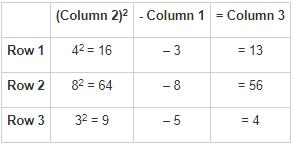Hence the missing number is 4.

QUESTION: 17

Some horses and same number of persons were going somewhere. Half of the persons were sitting on their horses whereas remaining persons were walking and leading their horses. If the number of legs walking is 70, then how many horses were there?

Solution:

Let,
Number of horses = x
Number of men = x
Number of walking men = x/2
Total legs of horses = 4x
Total legs of walking men = 2(x/2)
And,
2(x/2) + 4x = 70
5 * x = 70
X = 14

QUESTION: 18

If S means ‘+’, L means ‘×’, U means ‘÷’ and K means ‘-‘, then the value of
21 S 1 U 7 L 15 U 6 L 14 K 55 is

Solution:

Substituting the symbols:
21 + 1 ÷ 7 × 15 ÷ 6 × 14 - 55
Solution through BODMAS:
21 + 1/7 × 15/6 × 14 – 55
21 + 1/7 × 5/2 × 14 - 55
21 + 5 - 55
-29

QUESTION: 19

Six people A, B, C, D, E and F are sitting on the ground in a hexagonal shape. All the sides of the hexagon so formed are of the same length. A is not adjacent to B or C. D isn’t adjacent to C or E; B and C are adjacent, F is in the middle of D and C.
Question: Who among the following are not neighbours?

Solution:

People: A, B, C, D, E and F.
1. B and C are adjacent. ⇒ This gives us two possibilities: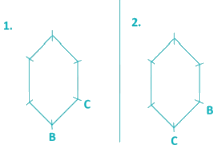2. F is in the middle of D and C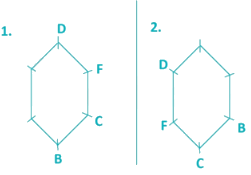3. A is not adjacent to B or C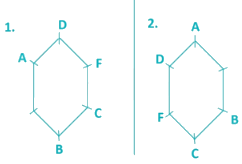4. D isn’t adjacent to C or E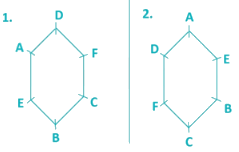Hence, two seating arrangements are possible.
Clearly, A & F aren’t neighbours in either of the cases.

QUESTION: 20

Directions: In the following question, among four answer figures whose cut pieces can form the given question figure.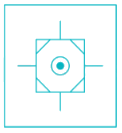Solution:

The given figure is made of:

1. An octagon
2. 4 triangular figures
3. 1 plain circle
4. 1 solid circles
5. 4 lines

These parts are available in option 2.

QUESTION: 21

From the given answer figures, select the figure which is hidden/embedded in the questions figure.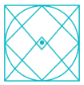Solution:

Clearly, this figure is hidden in the given figure as shown below,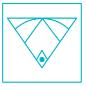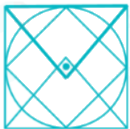QUESTION: 22

A piece of paper is folded and punched as shown below in the question figures. From the given answer figures, indicate how it will appear when opened.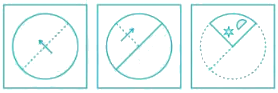Solution: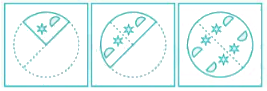When we will open piece of paper as it is folded then it will appear as it is shown in above diagram,
Therefore, image of option 2) is correct answer.

QUESTION: 23

One statement is given followed by two conclusions/assumptions, I and II. You have to consider the statement to be true, even if it seems to be at variance from commonly known facts. You are to decide which of the given conclusions/assumptions can definitely be drawn from the given statement. Indicate your answer.
Statements:
All Hindus are God fearing. No Japanese is a Hindu.
Conclusions:
I. The Japanese are not God fearing.
II. All God fearing are Hindus.

Solution: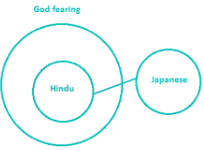From the above diagram, we cannot deduce a concrete relationship between God fearing and Japanese, so statement I is incorrect. We also see that not all God Fearing are Hindu, so statement II is also incorrect.

QUESTION: 24

Which of the answer figures is embedded in the question figure?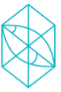Solution:

1) is not possible as there are no triangles with straight lines of this size.

2) is not possible as there are no right angled triangles in the given figure.

3) is seen in the diamond formed at the bottom by the two intersecting diagonals.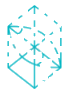4) is not possible as there is no curve intersected perfectly in half by a straight line.

QUESTION: 25

Which of the following answer figure patterns can be combined to make the question figure?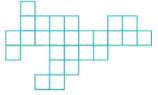Solution: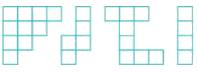Therefore image in option 4) can be combined to get the question figure.